# OHM’S LAW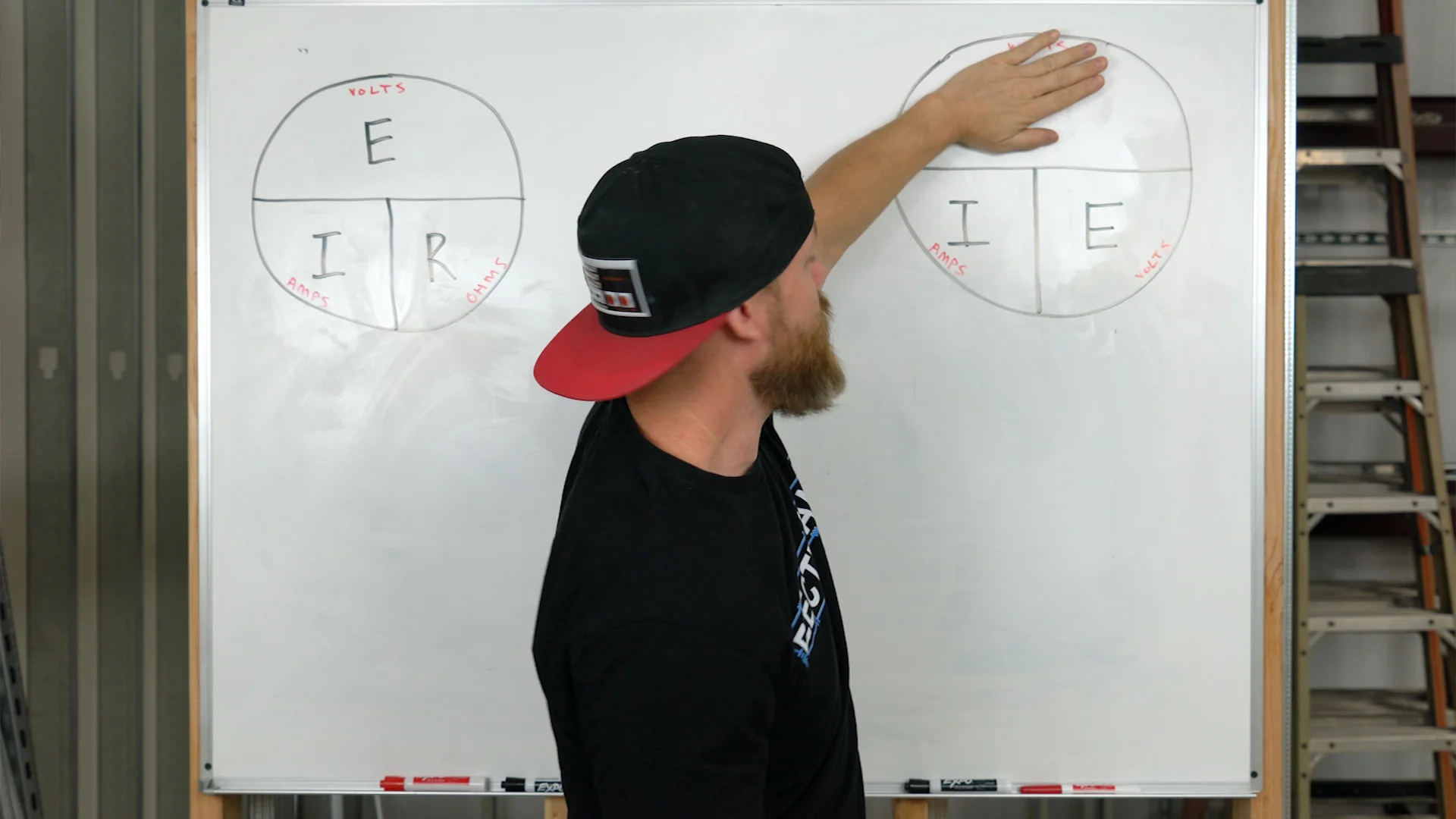First off, what is ohm’s law?  The short answer is a relationship.  It’s a relationship that exists between electrical units of measure, chiefly volts, amps, ohms, and watts.  Each of these values have a nearly perfect mathematical interactions, and when you start to raise or lower one, the others change to keep the relationship balanced.

This becomes extremely useful when you’re troubleshooting and need to find a missing value.  You can take readings in your environment to find some knowns, which you can then plug into an equation and do some simple math to solve for any unknowns.  Ok enough talking about it, let’s look at a few examples:

# LIGHTS ON A CIRCUIT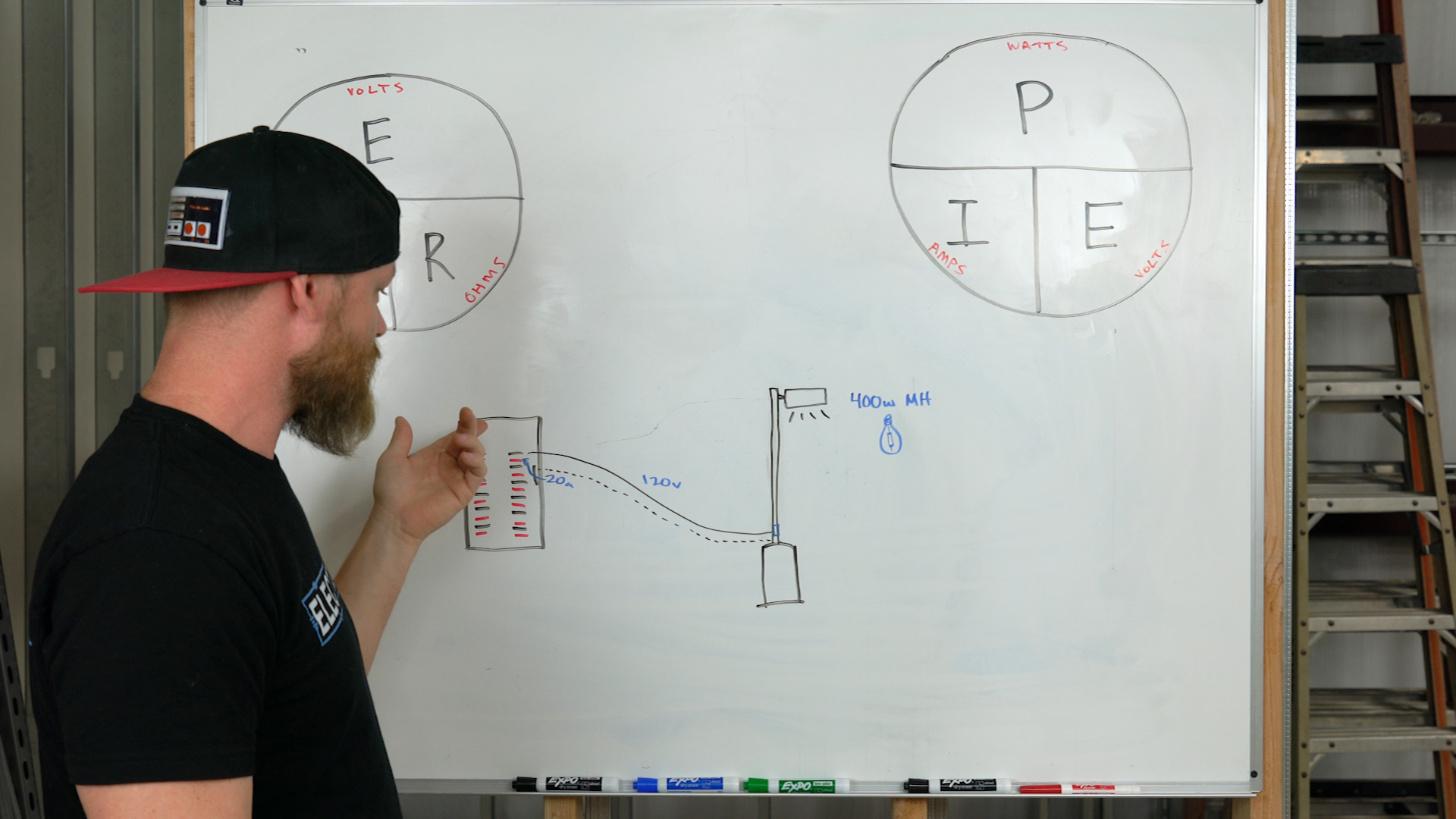One thing we do often as electricians is figure out how many light-fixtures we can install on a circuit.  Too many and we’ll keep tripping breakers.  This one’s easy so pull out a pencil and paper and do this as I go through it.

We have a 120-volt, 20-amp circuit with that was run out to a parking lot for pole-lighting.  Each pole has a 400-watt MH lamp installed in it. It appears 9 light fixtures were installed on this circuit, and the breaker keeps tripping.  Time to do math.

Start with the knowns.  We know we have volts, amps, and watts right?  We also know each fixture consumes a certain amount of wattage, and when we multiply volts and amps together we get wattage.  So 120 x 20 = 2,400 total watts available on this circuit.  And we know each lamp is 400w.

Now the unknowns. So we could divide 2,400 total circuit watts by 400 watts per lamp and find that there should only be 6 lamps on this circuit.  3 less than how it was wired.  We can also figure out how many watts the 9 existing 400w MH lamps are consuming.  Simply take 9 x 400 = 3,600 watts.  3,600 watts can also be expressed in amperage if we divide 3,600 by 120-volts – which comes out to 30-amps.  This means the existing circuit with 9 lamps on it, is drawing 30 amps as it’s consuming 3,600 watts of energy!  This will trip a 20-amp breaker any day of the week.  We would either need to drastically reduce the wattage of each lamp, re-pull larger conductors AND change the breaker to a larger breaker, or cap a few of these poles off and reduce the number that are allowed to turn on.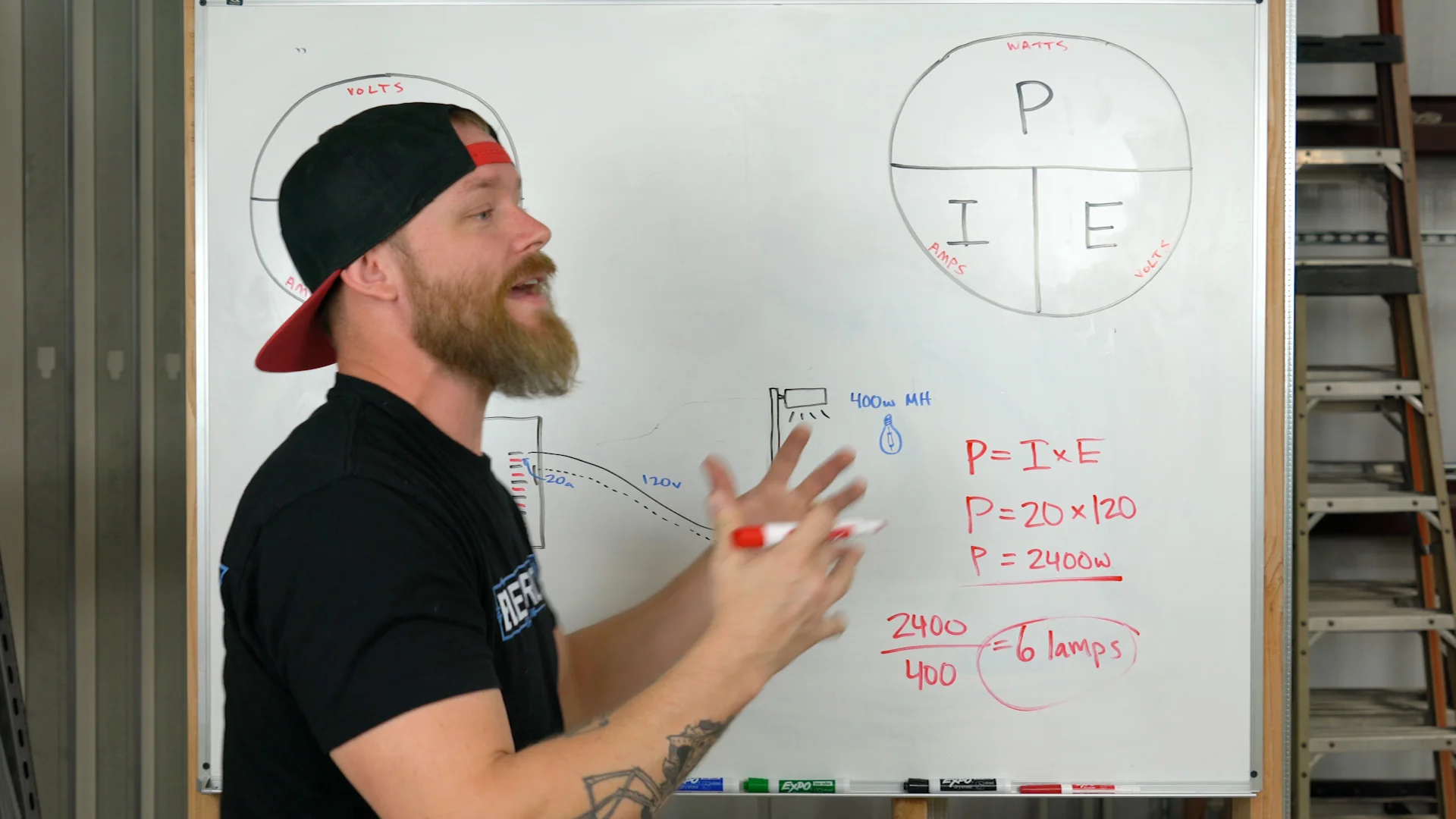For the above example, I used simple math so we could hack through the concept.  However, we need to think about this example one step further.  Since this is a commercial property the chances for these lights to stay on for 3 or more hours at a time, makes them a continuous load.  Breakers for continuous loads should not be loaded up to more than 80% of the circuit rating.  This means that rather than loading 20-amps of lighting on a 20-amp breaker is too much.  We really need to multiply that 20-amps by 80% (0.8) to get 16-amps.  If we can only run 16-ams of current on this circuit, that also changes the wattage.

For example, we take the same scenario in the example above.  120-volts, 400-watt lamps, but instead of a 20-amp circuit – we use 16-amps for this continuous load.  120 x 16 = 1920 total circuit watts.  1920 / 400 = 4.8 fixtures.  So in essence, a 20-amp circuit with continuous lighting loads on it should only have 4 400-watt fixtures on it.

# FINDING WATTAGE OF A LAMP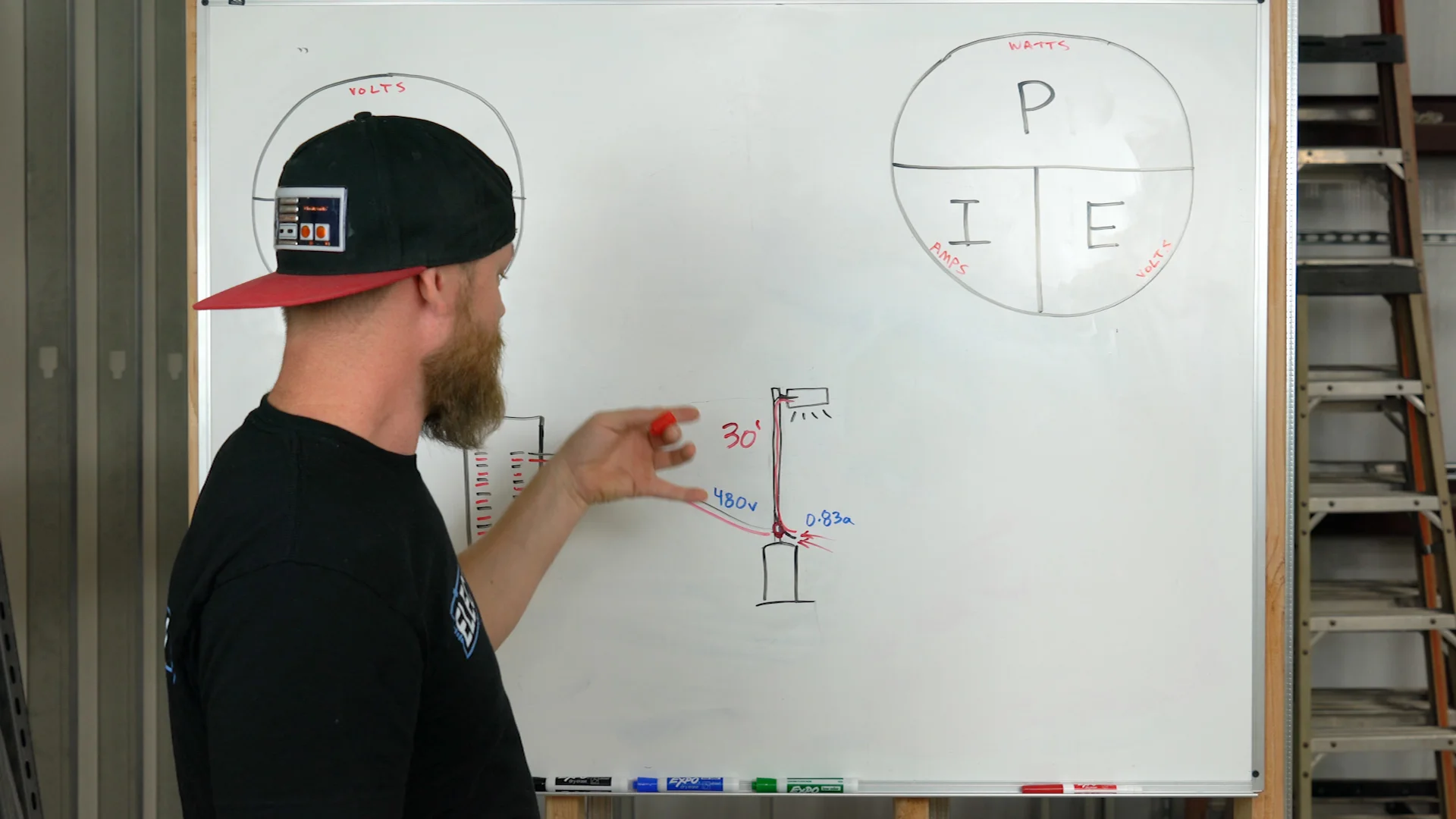One impressive trick to pull out when you’re in a parking lot doing pole-lights is to find the wattage of a lamp that’s 30-ft in the air without even getting up in a ladder or a bucket truck.  Every pole has a hand-hole with wires in it.  So this means we have an opportunity to test for values.  Every lamp or bulb is rated in wattage, so we know we’re trying to find what the wattage of that lamp is using other values.

For this, the circuit needs to be on or nothing will work.  First you test voltage, and say in this example we test 480-volts, then we test the amperage flowing through the conductors and test 0.83-amps.  Well as you may have guessed by now, using P = I x E (mmmmmm, PIE, yummm).  This works out to P = 480 x 0.83, or 400-watts.  Now you can tell if it’s metal halide, mercury vapor, or high-pressure sodium just by looking at the shape and characteristics of the lamp but at least now you know what wattage you’re working with so you can order lamps and ballasts without having to have the bucket truck around just to gather information.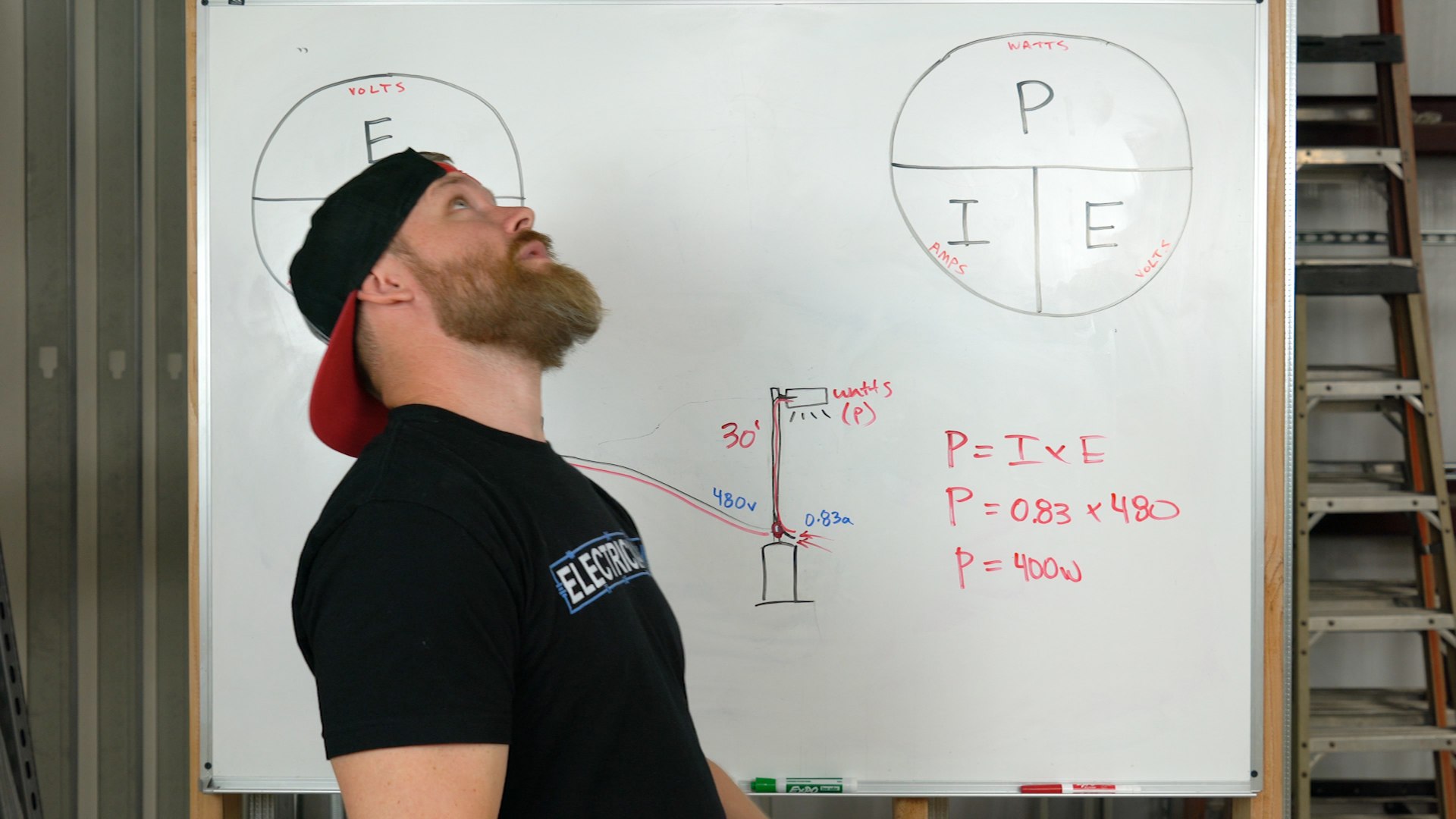# VOLTAGE-DROP (Ohm’s Law version)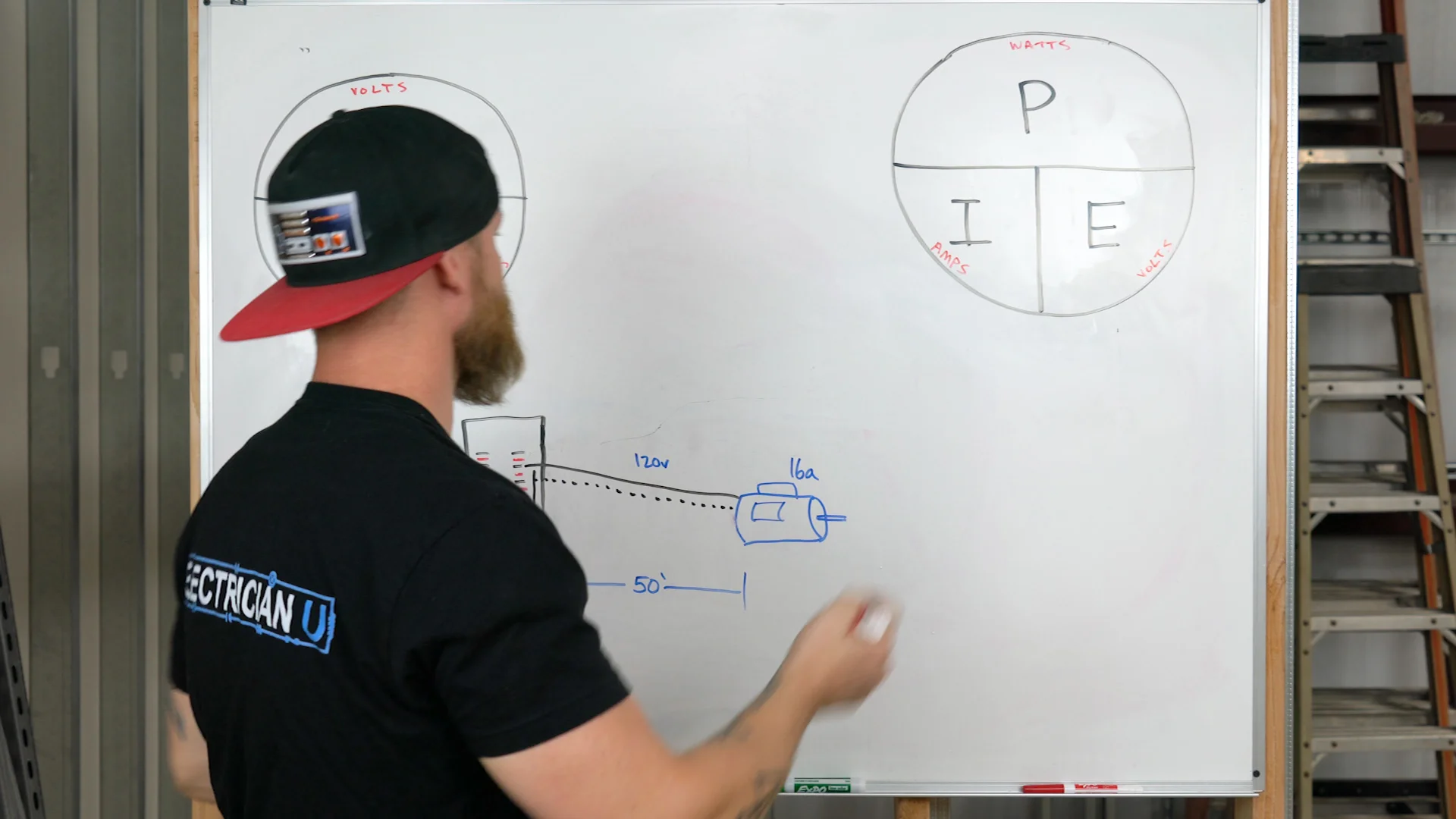Most people are used to using a specific formula to calculate voltage-drop:

Vd = 2KID/Cm

This is a great calculation to use, however it can take time to find the values needed to plug into this formula.  A quicker way is to use ohm’s law to get a very similar answer.

Ed = I x R

Here’s our example.  We have a 16-amp motor on a job that is 50 feet away from the panel it’s wired to.  This motor’s nameplate says that it is a 120-volt single-phase rated motor and we’re running #12 THHN to it.  So we have a lot of values to start plugging in.  We’re trying to find voltage-drop, not voltage itself.  We want to know how much is the voltage of this circuit going to drop once this motor kicks on.

For that we take Ed = I x R and plug in 16-amps for the current, but how do we know the resistance of the circuit to plug in for R?  We can look in the NEC or in an Ugly’s manual to find out what the resistance values of specific conductors are per 1000 ft. #12 stranded has 2-ohms of resistance per 1,000 ft, which also means it has 0.2 ohm’s per 100 ft.  But wait don’t we want 50 ft? No.  Over 50 ft we have 2 conductors, so really current has to travel down both of them to complete the circuit.  So we wan’t the 100 ft value of 0.2 ohms.

All that said, now we have Ed = 16 x 0.2, which means the voltage drop of this circuit ends up being 3.2-volts.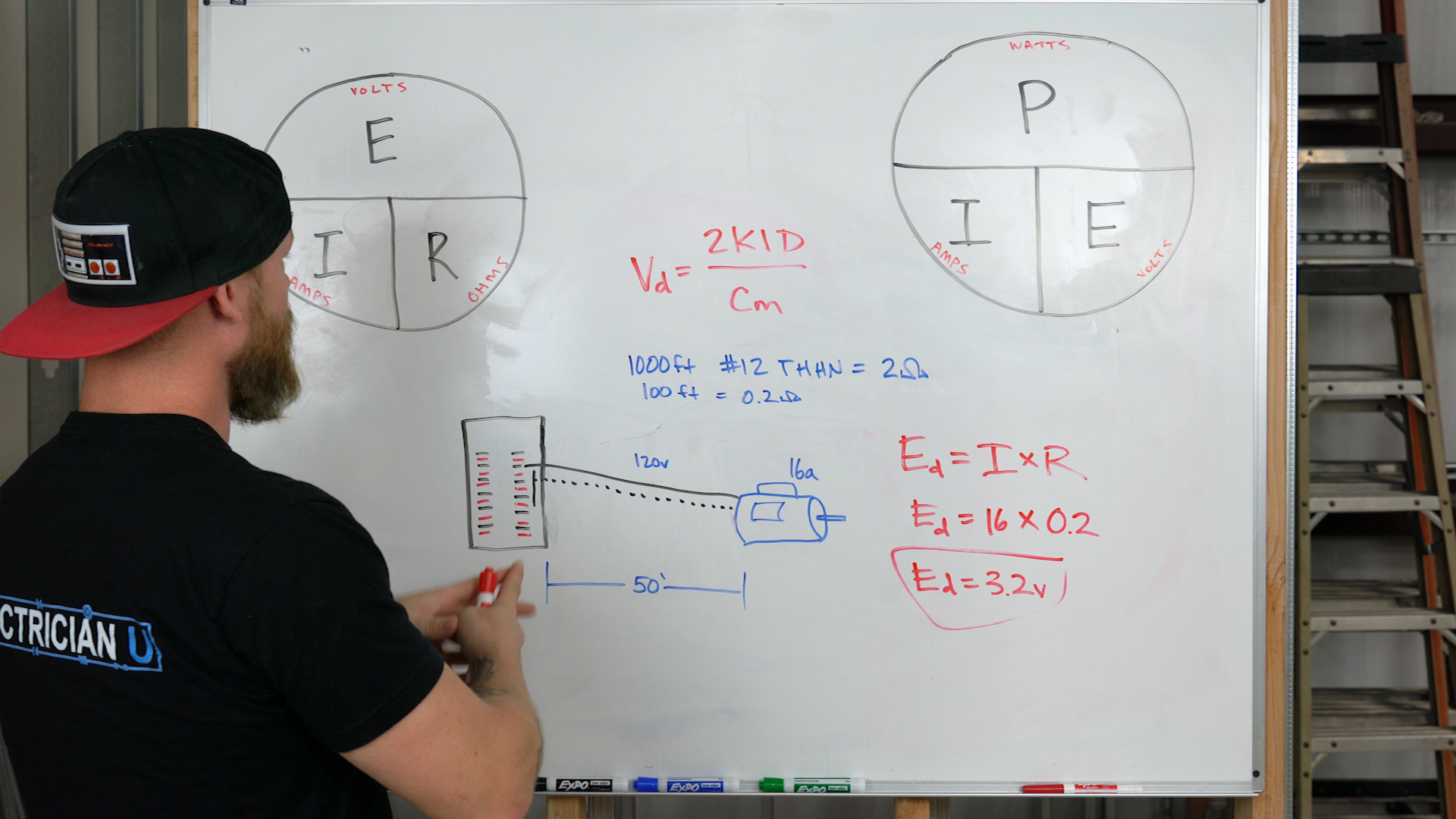Now we can still use the more popular voltage-drop formula Vd = 2KID/Cm but here we have to have memorized K values for copper and aluminum, we need to remember not to double the distance, rather treat the run as if it were just one conductor, and we’d need to look up the circular mill value of #12 stranded wire.  Not really all that difficult but more steps than just using ohm’s law.  The values you’ll get are very similar.

One last thing to note on this is that when you have a 3-phase load you cannot use the ohm’s law method.  You’d actually use 1.732KID/Cm to solve for this and there’s no formula I know of with ohm’s law to calculate 3-phase voltage drop.  Bummer.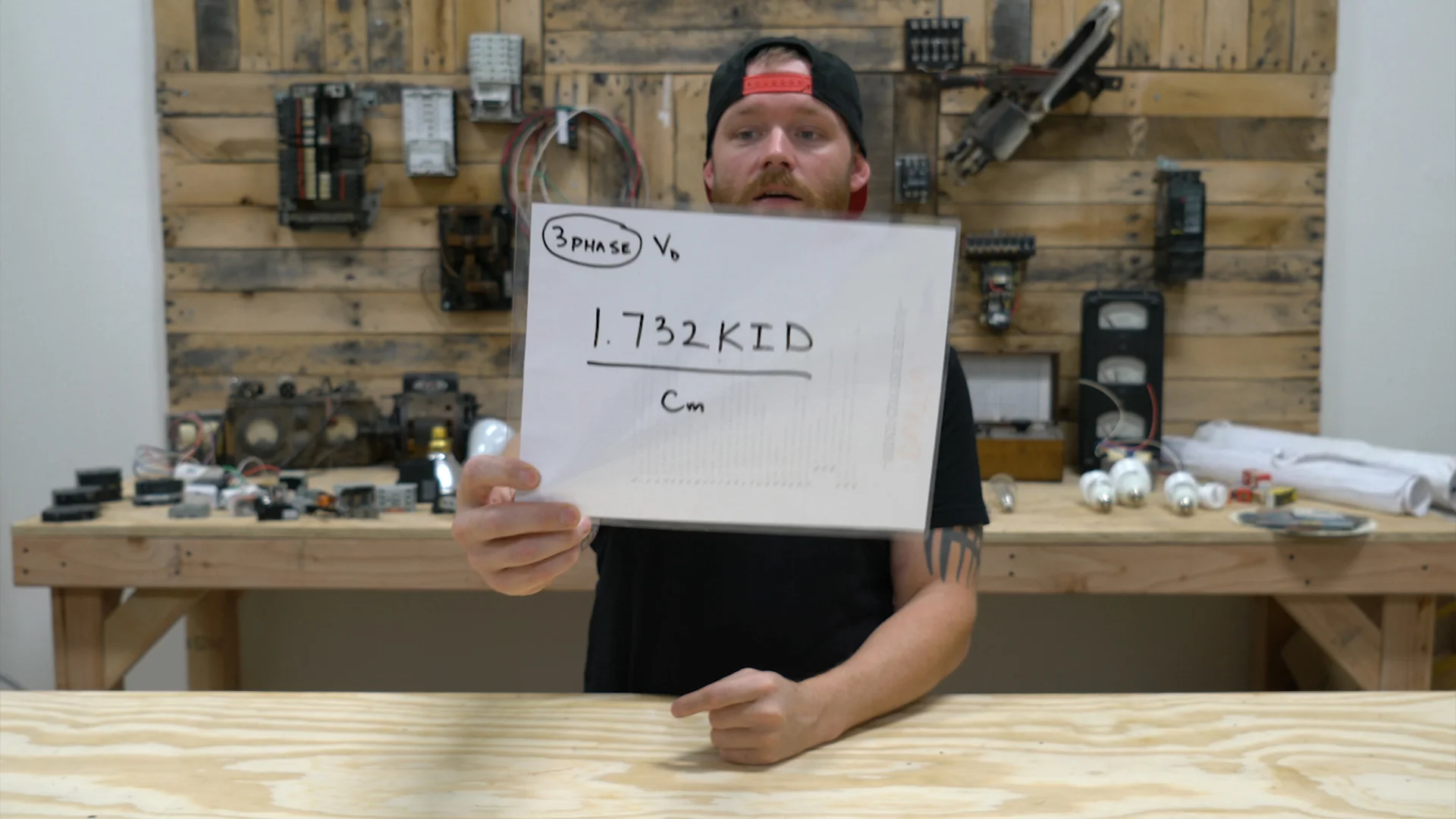# HEATING ELEMENTS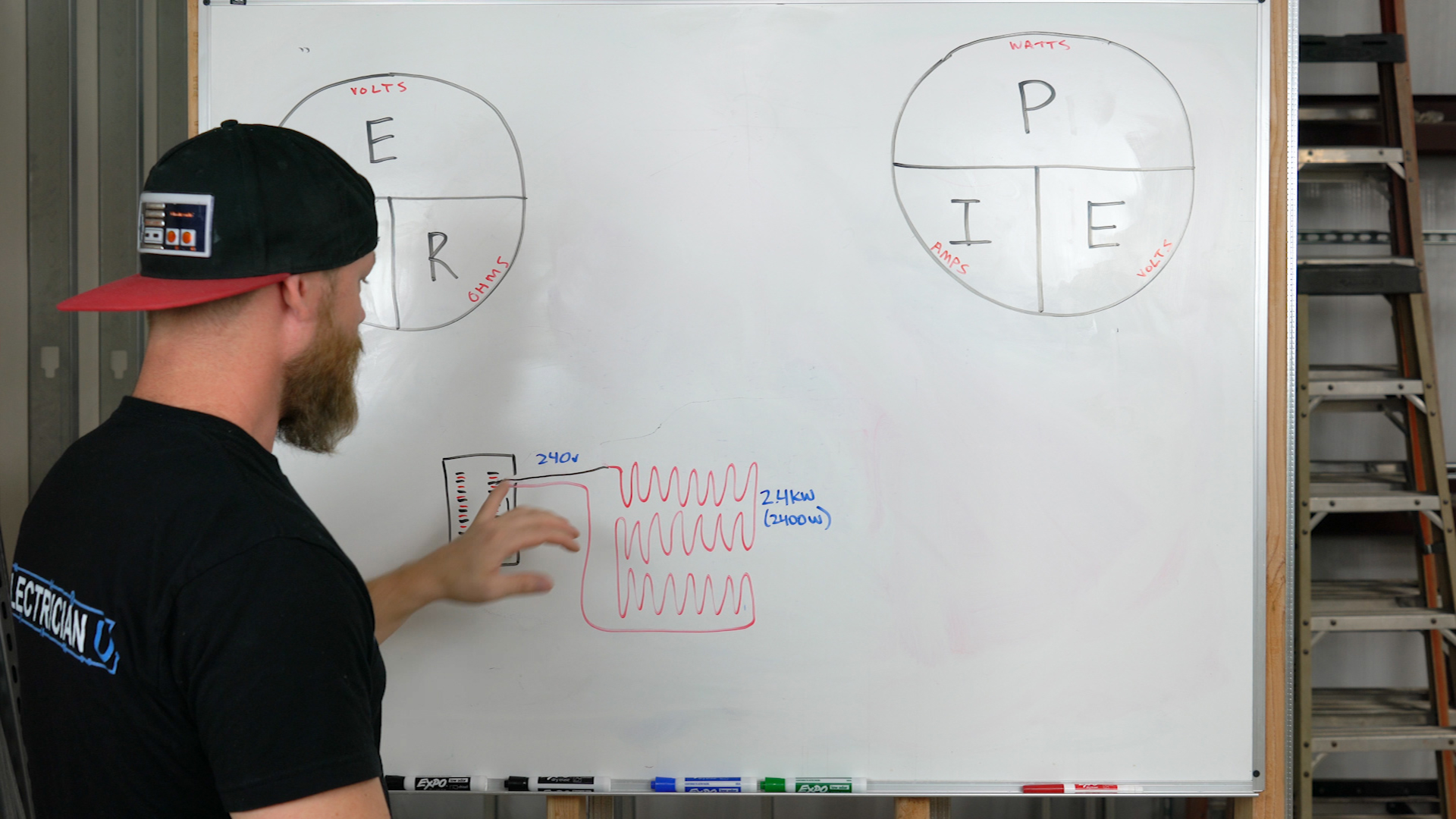Say we have an appliance that has a heating element run through it.  We see on the nameplate of the appliance that this is a 240-volt, 2400-watt element.  How do we figure out what conductors to run to the appliance?  Well for conductor sizing we need to know the amp-draw of the circuit.  So we look at our equations and see that we have volts and watts, and need to come up with amps.  So we put together a little equation E x I = P stating 240 x A = 2400.  We need to get A by itself, so we divide both sides of the equation by 240 and get A=10-amps.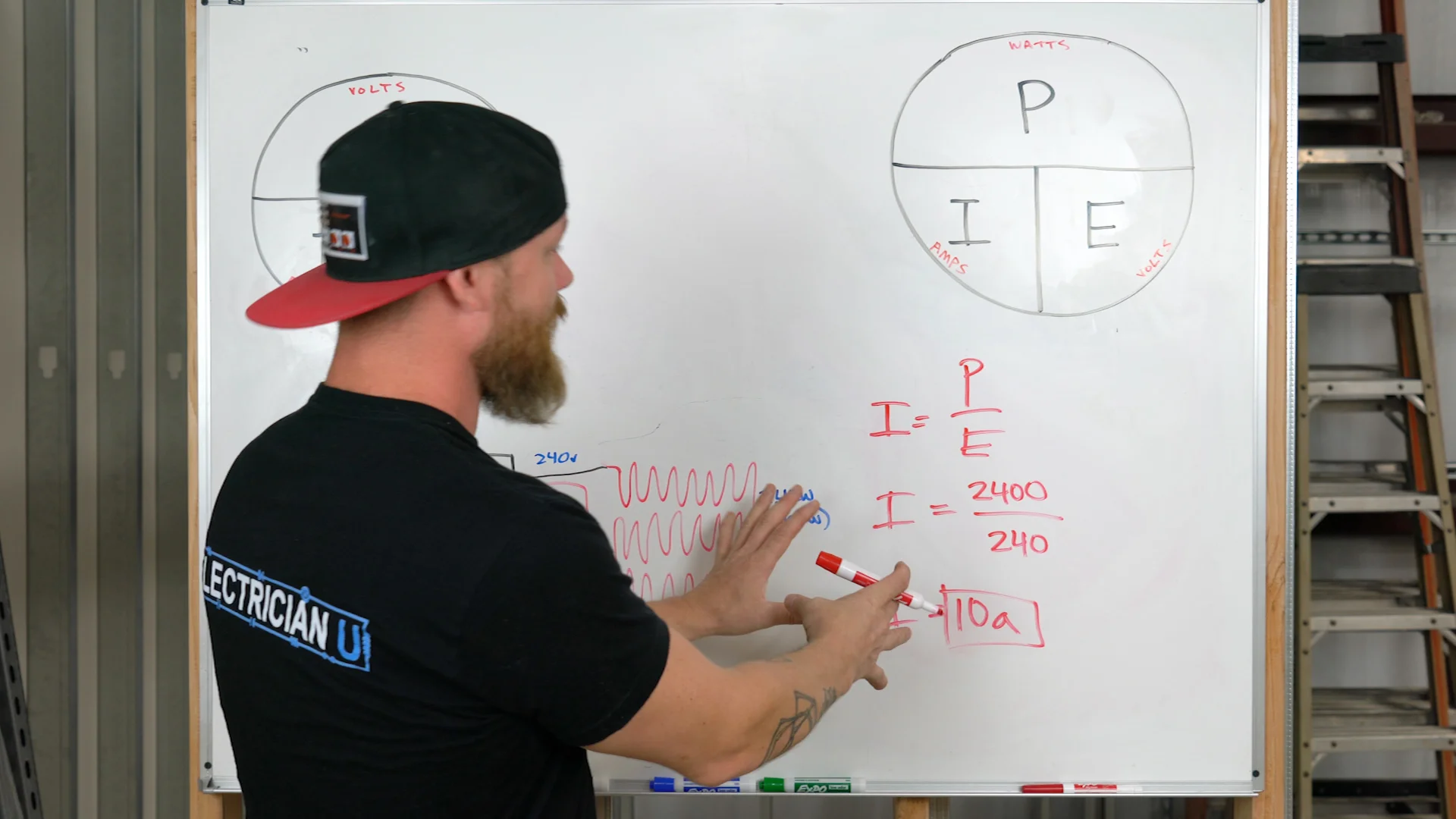We could have done this another way as well.  Say that we didn’t have the wattage rating of this element but we knew for sure that we had 240-volts run to it.  We could take our multi-meter out and test for the resistance of the element from one end to the other.  Then we can take this resistance value and plug it in as a known with the voltage.  Say we tested resistance and found 24-ohms.  Still looking for Amps here.  So E = I x R is the relationship we’re looking for.  240 = I x 24, which makes I still equal 10 amps.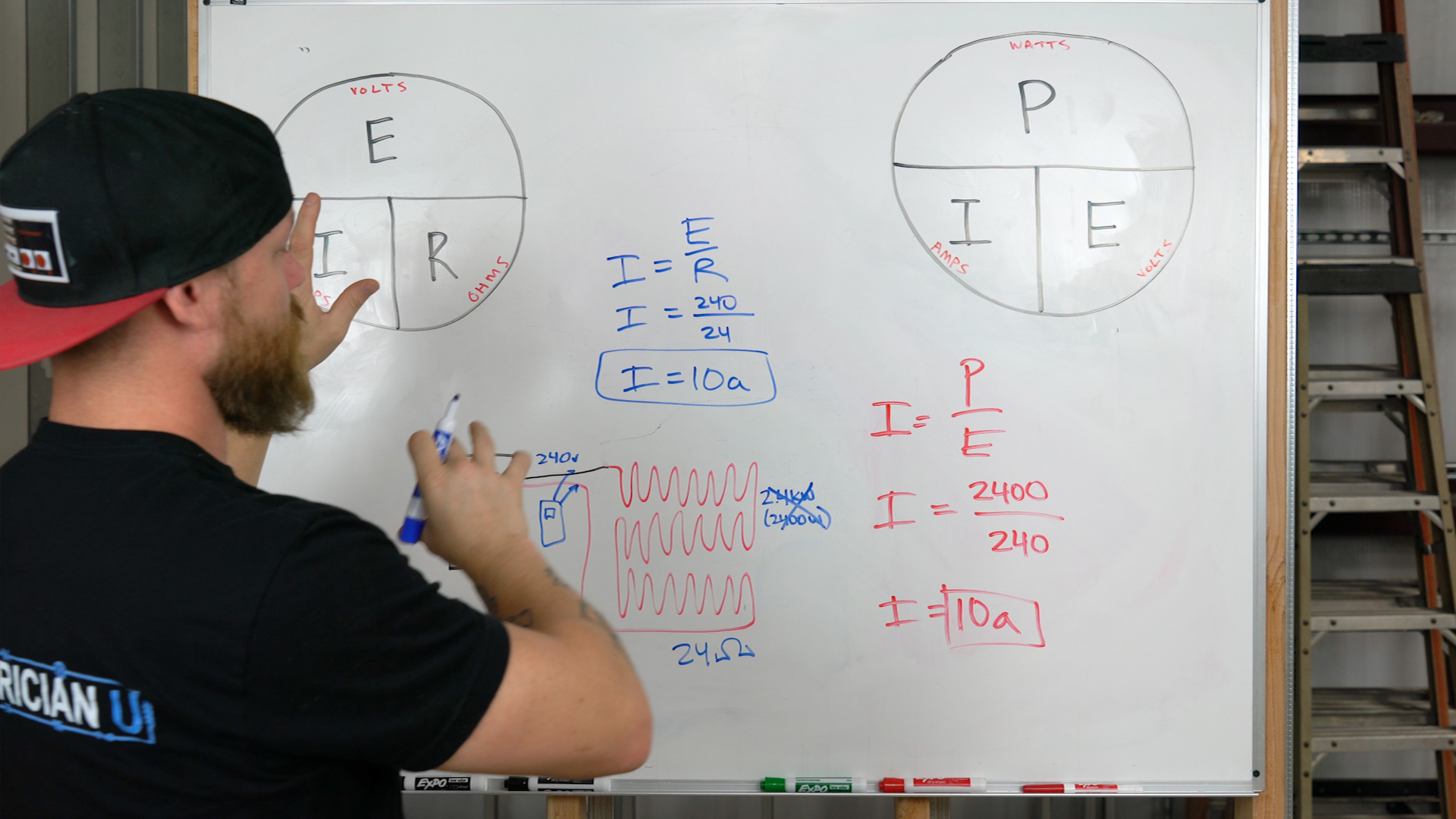# HOW EQUATIONS WORK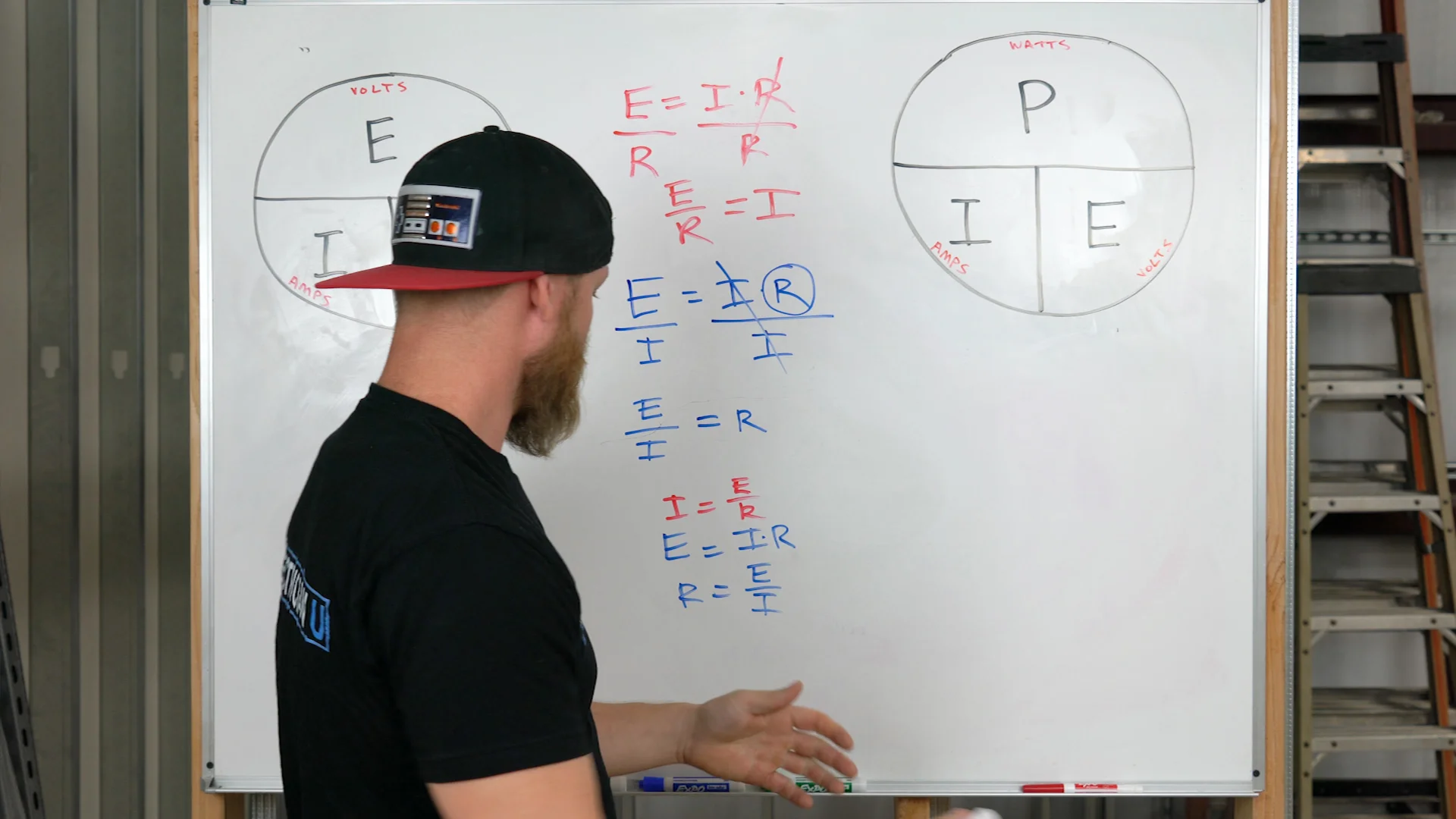Equations are not very difficult to work with.  All you need to understand is that both sides of the equal sign, have to….well…equal.  An equation is a statement.  P = I x E is really saying the wattage of the circuit is equal to the current flowing times the voltage doing the pushing/pulling.  But what if we’re not solving for P?  What if we need to solve for I, but all we can remember is PIE. (P = I x E). Well we can isolate I  by dividing both sides of the equation by E.  This deletes E on the IxE side, and moves it under the P on the other side of the equation – giving us a new equation of P / E = I.  This equation is staying the same thing as P = I x E, but it allows you to find a different missing value.  Same thing works with isolating E, just divide both sides of P = I x E by I – giving you P / I = E

Once you understand how to rearrange equations and get plug in values, this will all make more sense to you.  It does however take practice to get there.  So work this stuff with a pencil and paper often.  Take the values that you have around you on these jobs and try to calculate things that you don’t know.  It will get you understanding things a little easier from now on.

## Please check out the Electrician U Facebook page and subscribe to theYouTube Channel if you like these videos! Also check out the Electrician U Instagram page.

**Disclaimer – These videos are for training purposes alone, all work done on electrical systems should be done by a licensed and insured electrical contractor.  If you are not an electrician, do not attempt any of the work you are seeing in these videos.**